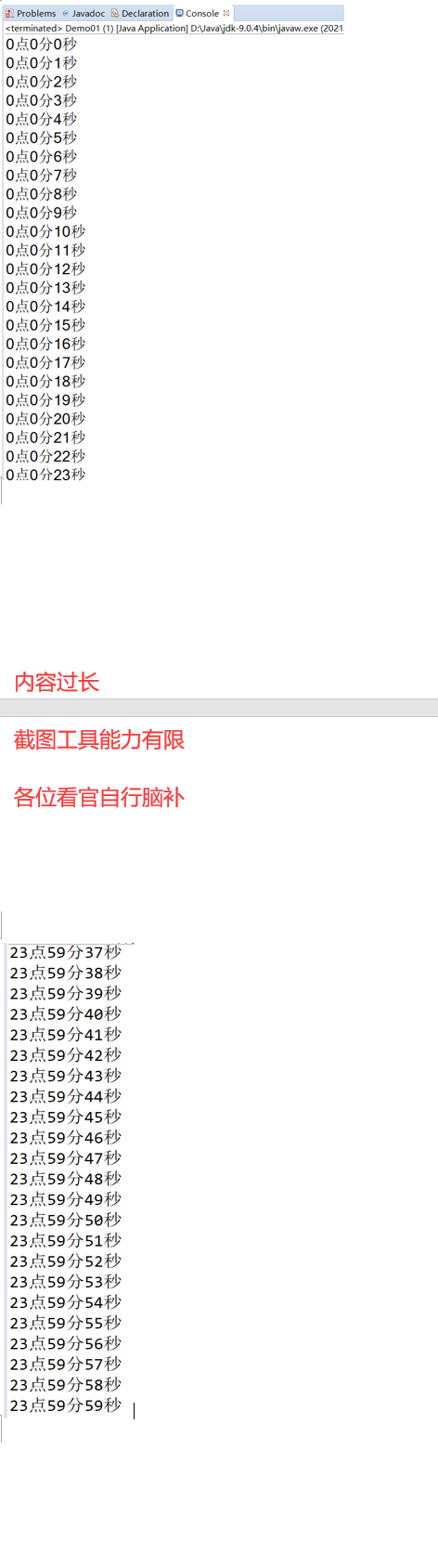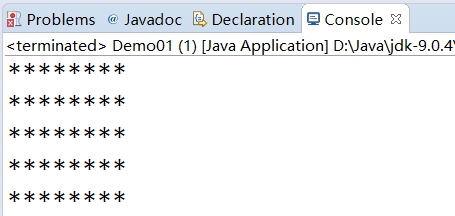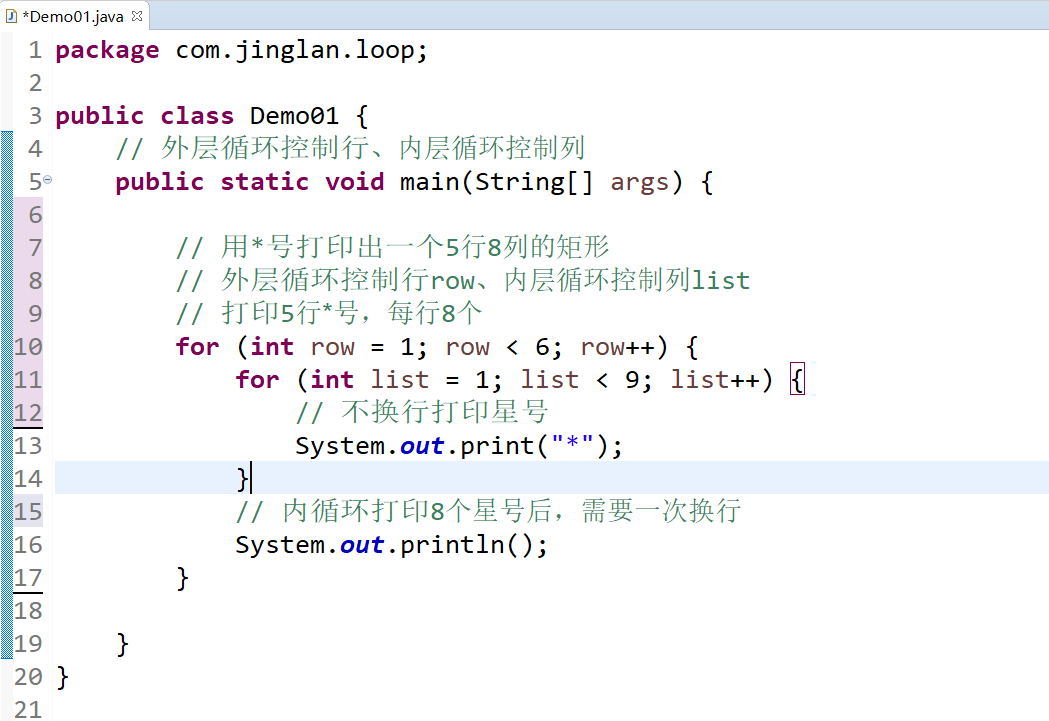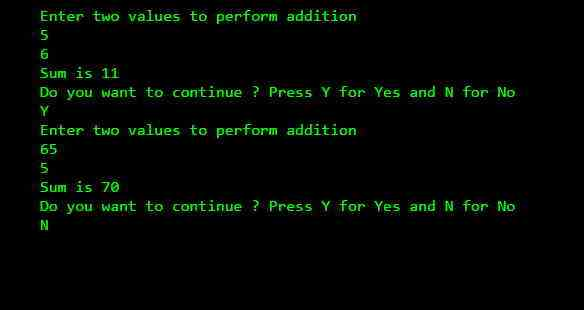• 嵌套循环 所谓嵌套循环，是指一个循环的循环体是另一个循环。比如for循环里面还有一个for循环，就是嵌套循环。 **总共的循环次数=外循环次数 * 内循环次数 外层循环执行一次、内层循环执行多次 ** ...
嵌套循环
所谓嵌套循环，是指一个循环的循环体是另一个循环。比如for循环里面还有一个for循环，就是嵌套循环。
总共的循环次数=外循环次数 * 内循环次数 外层循环执行每一次、内层循环就会执行多次
【例题1】循环输出时、分、秒 1小时60分钟，1分钟60秒
package com.jinglan.loop;

public class Demo01 {

public static void main(String[] args) {
for (int hour = 0; hour < 24; hour++) {// 小时
for (int minute = 0; minute < 60; minute++) {// 分钟
for (int second = 0; second < 60; second++) {// 秒
System.out.println(hour + "点" + minute + "分" + second + "秒");
}
}
}
}
}

运行结果 太长了！从0时0分0秒到23时59分59秒温馨提示：你可能会出现代码运行后的控制台上数据显示不全的问题，那是因为控制台显示数据的长度收到了限制 解决方法：Window->Preferences->Run/Debug->Console->取消Limit console output前的复选框。
【例题2】打印出一个5行8列的矩形 外层循环控制行、内层循环控制列
package com.jinglan.loop;

public class Demo01 {
// 外层循环控制行、内层循环控制列
public static void main(String[] args) {

// 用*号打印出一个5行8列的矩形
// 外层循环控制行row、内层循环控制列list
// 打印5行*号，每行8个
for (int row = 1; row < 6; row++) {
for (int list = 1; list < 9; list++) {
// 不换行打印星号
System.out.print("*");
}
// 内循环打印8个星号后，需要一次换行
System.out.println();
}

}
}


运行结果：对于【例题2】这里做一个详细的执行顺序的解说从第一个for循环开始执行——》int row = 1；——》row<6满足条件，执行循环体，首先执行循环体中的for循环——》int list=1;——》list<9满足条件，执行System.out.print("");语句生成一个 星号，执行——》list++；此时list=2，依旧满足list<9的循环条件， 执行System.out.print("");语句生成第二个星号…生成第八个星号，执行——》llist++，此时list=9，不满足循环条件，于是内层循环执行结束，程序向下执行System.out.println();语句生成一个空行，后续没有需要执行的代码了，于是row++，此时row=2满足外层循环条件，于是外层for循环继续向下执行——》int row = 2；——》row<6满足条件，执行循环体，首先执行循环体中的for循环——》int list=1;——》list<9满足条件，执行System.out.print("");语句生成一个 星号，执行——》list++；此时list=2，依旧满足list<9的循环条件，执行System.out.print("");语句生成第二个星号…生成第八个星号，执行——》llist++，此时list=9，不满足循环条件，于是内层循环执行结束，程序向下执行System.out.println();语句生成一个空行，后续没有需要执行的代码了，于是row++，此时row=3满足外层循环条件， … 外层循环结束，完美运行出结果
【例题3】使用循环语句打印出一个10行10列的星号 跟【例题2】原理一样，改变一下数字即可
package com.jinglan.loop;

public class Demo01 {
// 外层循环控制行、内层循环控制列
public static void main(String[] args) {

// 打印出一个10行10列的星号
// 外层循环控制行row、内层循环控制列list
// 打印10行*号，每行10个
for (int row = 1; row < 11; row++) {
for (int list = 1; list < 11; list++) {
// 不换行打印星号
System.out.print("*");
}
// 内循环打印个10星号后，需要一次换行
System.out.println();
}

}
}


展开全文• c语言 循环嵌套循环 Here you will learn about nested loops in C. Nesting is one of the most complex topics of C programming. Just like decision control instructions, a loop control instruction can ...c语言 循环嵌套循环

Here you will learn about nested loops in C.
在这里，您将了解C中的嵌套循环。
Nesting is one of the most complex topics of C programming. Just like decision control instructions, a loop control instruction can also be nested easily. But sometimes understanding the execution of such programs could be a bit tricky. So it is important to make the basics clear.
嵌套是C编程中最复杂的主题之一。 就像决策控制指令一样，循环控制指令也可以轻松嵌套。 但是有时了解此类程序的执行可能会有些棘手。 因此，弄清楚基础知识很重要。
C中的嵌套循环 (Nested loops in C)
As I said in my earlier tutorials, nesting means defining statement under the scope of another similar statement. In case of loops, when we nest two loops then it generally multiplies the execution frequency of loops.
正如我在之前的教程中所说的，嵌套意味着在另一个类似语句的范围内定义语句。 在循环的情况下，当我们嵌套两个循环时，它通常会乘以循环的执行频率。
We can nest for loop inside while loop and vice versa is also true. Generally programmer nest up to 3 loops. But there is no limit of nesting in C.
我们可以在循环内嵌套for循环，反之亦然。 通常，程序员最多嵌套3个循环。 但是在C中嵌套没有限制。
Now let’s try a small program with nested loops.
现在让我们尝试一个带有嵌套循环的小程序。
#include<stdio.h>

void main()
{
int row,col;
for(row=1;row<4;row++)
{
for(col=1;col<4;col++)
{
printf("%d\t%d\n",row,col);
}
}
}
Output
输出量Explanation
说明
Every row executes a column at least 3 times. Therefore you are seeing all three values with every row. 每行执行一列至少3次。 因此，您将在每一行看到所有三个值。 Once the control reaches inside the inner loop, it came out only when the loop is fully executed. 一旦控件到达内部循环内部，则仅在循环完全执行后才出现。 We cannot alter the control once it reaches inside the inner loop. 一旦控件进入内部循环，我们将无法对其进行更改。
Note: I have deliberately shown a simple program to show nesting of loops. Execution of nested loops can’t be summarized in words. So it will be better if you try your hands on the nesting of loops to understand them better.
注意：我故意显示了一个简单的程序来显示循环嵌套。 嵌套循环的执行不能用文字概括。 因此，如果您尝试循环嵌套以更好地理解它们，那就更好了。
奇数循环 (Odd Loop)
There are situations when we need to execute a set of instructions until the user deny it. So we need to check if the user wants to repeat the set of instructions or not.
在某些情况下，我们需要执行一组指令，直到用户拒绝为止。 因此，我们需要检查用户是否要重复这组指令。
In that case programmer has no idea about the executing time and frequency of the program. This is called odd loop in C programming. So basically we have to make a program which will ask to the user about the re-execution of program.
在那种情况下，程序员不知道程序的执行时间和频率。 这在C编程中称为奇数循环。 因此，基本上我们必须制作一个程序，该程序将询问用户有关程序的重新执行。
#include<stdio.h>

void main()
{
char yes='Y';
int x,y;

while(yes=='Y')
{
printf("Enter two values to perform additionn");
scanf("%d%d",&x,&y);
printf("Sum is %d n",x+y);
printf("Do you want to continue ? Press Y for Yes and N for Non");
scanf("%c", &yes);
}
}

Output
输出量Explanation
说明
In the beginning I have character variable yes with character initial value ‘Y’. After that I have declared two more integer variables. 一开始，我有字符变量yes，字符初始值为“ Y”。 之后，我又声明了两个整数变量。 Now I have started while loop with condition yes==’Y’. It means the loop will be executed until it receives value ‘Y’. 现在，我以条件yes =='Y'开始了while循环。 这意味着循环将一直执行，直到收到值“ Y”为止。 After that I have displayed the message to enter two values. By using scanf() function I have stored data inside the variables. 之后，我显示了输入两个值的消息。 通过使用scanf()函数，我将数据存储在变量中。 Now instead of using a third variable to calculate sum. I have used a simple argument to calculate sum inside printf() function. 现在，而不是使用第三个变量来计算总和。 我使用了一个简单的参数来计算printf()函数中的总和。 In the end the program will display the message for user. If he wants to continue then he will press “Y”. Otherwise he will press “N” and the program will be terminated. 最后，程序将为用户显示消息。 如果他想继续，那么他将按“ Y”。 否则，他将按“ N”，程序将终止。
Comment below if you have doubts or found any information incorrect in above tutorial for nested loops in C.
如果您有疑问或在上面的教程中对C中的嵌套循环有任何不正确的信息，请在下面评论。

翻译自: https://www.thecrazyprogrammer.com/2015/01/nested-loops-in-c.html

c语言 循环嵌套循环

展开全文java python c语言 编程语言 c++
• shell编程之随机数和嵌套循环 文章目录shell编程之随机数和嵌套循环一、随机数1. 如何生成随机数？2. 实战案例二、嵌套循环三、expect 一、随机数 关键词：一切都是未知数，永远不知道明天会抽什么风
• 本篇文章主要介绍了nodejs中解决异步嵌套循环和循环嵌套异步的问题，具有一定的参考价值，有兴趣的可以了解一下
• ## python：嵌套循环

万次阅读 多人点赞 2019-01-19 14:44:07
2、嵌套循环简单的来说就是一个循环出现在另一个循环里面。对于外循环的每次迭代，内循环都要完成它的所有迭代 while 循环嵌套 语法： while expression: while expression: statement(s) statement(s) 1...
Python循环嵌套
1、Python语言允许在一个循环体里面嵌入另一个循环
2、嵌套循环简单的来说就是一个循环出现在另一个循环里面。对于外循环的每次迭代，内循环都要完成它的所有迭代
3、当2个(甚至多个)循环结构相互嵌套时，位于外层的循环结构常简称为外层循环或外循环，位于内层的循环结构常简称为内层循环或内循环

循环嵌套类型
可以在循环体内嵌入其他的循环体，如在while循环中可以嵌入for循环， 反之，也可以在for循环中嵌入while循环     1、while循环中嵌套while    (常用)     2、for循环中嵌套for    (常用)     3、while循环中嵌套for循环     4、for循环中嵌套while循环

while循环嵌套
语法：
while expression:
while expression:
statement(s)
statement(s)
例1：使用while循环来实现乘法表
row = 1
while row <= 9:
col = 1
while col <= row:
print("%d*%d=%d\t" % (row, col, row*col), end = "")
col += 1
print() # 单纯的换行
row += 1

"""
1*1=1
2*1=2    2*2=4
3*1=3    3*2=6    3*3=9
4*1=4    4*2=8    4*3=12    4*4=16
5*1=5    5*2=10    5*3=15    5*4=20    5*5=25
6*1=6    6*2=12    6*3=18    6*4=24    6*5=30    6*6=36
7*1=7    7*2=14    7*3=21    7*4=28    7*5=35    7*6=42    7*7=49
8*1=8    8*2=16    8*3=24    8*4=32    8*5=40    8*6=48    8*7=56    8*8=64
9*1=9    9*2=18    9*3=27    9*4=36    9*5=45    9*6=54    9*7=63    9*8=72    9*9=81
"""
注： 1、循环嵌套结构的代码，Python解释器执行的流程为：     ⑴当外层循环条件为True时，则执行外层循环(属于外循环的语句)     ⑵外层循环体中包含了普通程序和内循环，当内层循环的循环条件为True时则执行内循环，直到内层循环条件为False，跳出内循环     ⑶如果此时外层循环的条件仍为True，则返回第2步，继续执行外层循环体，直到外层循环的循环条件为False     ⑷当内层循环的循环条件为 False，且外层循环的循环条件也为 False，则整个嵌套循环才算执行完毕
2、即：外部循环满足条件后，开始执行属于外循环的内部循环，等内部循环全部执行完毕，如果还满足外部循环条件，则外部循环再次执行，依次类推，直到跳出外层循环
3、python中的print打印函数默认自带换行，可以添加第二参数 end = " " 来阻止换行。end代表要打印字符串的最后最后一个字符，可以换成制表符或者其他的符号代替换行符而已
例1_1：
#while循环求1-10内的素数
i = 2
while (i < 10):
j = 2
while j <= (i / j):
if not (i % j):
break
j = j + 1
if (j > i / j):
print(i, " 是素数")
i = i + 1

"""
2  是素数
3  是素数
5  是素数
7  是素数
"""
注： 1、在循环(while循环或for循环)中，可以使用continue或break语句来终止循环     ⑴continue或break语句用来终止循环语句，即循环条件没有False条件或者序列还没被完全递归完，也会停止执行循环语句     ⑵跳出当前循环，不会执行brea或continuek后面的代码：不会执行当前循环下所属代码块,但会继续执行下面其他代码块的代码(与循环语句同一缩进的代码)
2、如果使用嵌套循环，break语句将停止执行最深层的循环，并开始执行下一行代码
3、Python不支持一次跳出多个循环，我们可以通过在父循环中设定一个跳出标志，子循环通过更改这个标志来跳出多个循环
例2：
loop1 = 0
loop2 = 0

while True:
loop1 += 1
print("父循环：", loop1)
break_flag = False
while True:
loop2 += 1
if loop2 == 5:
break_flag = True
print("跳出子循环")
break       #跳出当前循环，不会执行break后面的代码(当前所属循环代码块)
print("子循环：", loop2)#该语句与break语句属于同一循环，因此执行内循环的break后，不会执行该代码
if break_flag == True: #这条语句与内循环while同一缩进(不在内循环内)，因此执行内循环的break语句后，也会执行该代码
print("跳出父循环")
break

# break语句：跳出本次循环(嵌套循环中只跳出一层循环)

"""
父循环： 1
子循环： 1
子循环： 2
子循环： 3
子循环： 4
跳出子循环
跳出父循环
"""
注： break和continue的用法给我的感觉就是，在循环里面加一个if的判断语句     ⑴当满足这个if的条件时执行break或continue语句(并不再执行其后面的语句)     ⑵当不满足这个if判断语句时执行其他语句(其他语句正常写：先写正常的语句，再在正常的语句中加一个if的判断条件来跳出循环)

for嵌套循环
1、for循环可以用来遍历某一对象     ⑴遍历：通俗点说，就是把这个循环中的第一个元素到最后一个元素依次访问一次
2、for循环和while循环     ⑴两者的相同点在于都能循环做一件重复的事情     ⑵不同点在于，for循环是在序列穷尽时停止，while循环是在条件不成立时停止
3、不管是嵌套for循环还是嵌套while循环：每执行外循环一次，都要等待内循环全部完成或中止,才继续接着外循环,如此反复,直到外循环完成
语法：
for iterating_var in sequence:
for iterating_var in sequence:
statements(s)
statements(s)


例3：
for i in range(4):
print("外循环遍历出来的值：",i)
for n in range(i):
print("内循环遍历出来的值：",n)

"""
外循环遍历出来的值： 0

外循环遍历出来的值： 1
内循环遍历出来的值： 0

外循环遍历出来的值： 2
内循环遍历出来的值： 0
内循环遍历出来的值： 1

外循环遍历出来的值： 3
内循环遍历出来的值： 0
内循环遍历出来的值： 1
内循环遍历出来的值： 2
"""


例4：
#for循环求素数
num=[]
for i in range(2,100):
for j in range(2,i):
if(i%j==0):
break
else:
num.append(i)
print(num)

"""
[2, 3, 5, 7, 11, 13, 17, 19, 23, 29, 31, 37, 41, 43, 47, 53, 59......]
"""
例4_1：
#使用for循环实现乘法表
for i in range(1, 10):
for j in range(1, i+1):
print("%d*%d=%d\t" % (i, j, i*j), end="")
print()

"""
1*1=1
2*1=2    2*2=4
3*1=3    3*2=6    3*3=9
4*1=4    4*2=8    4*3=12    4*4=16
5*1=5    5*2=10    5*3=15    5*4=20    5*5=25
6*1=6    6*2=12    6*3=18    6*4=24    6*5=30    6*6=36
7*1=7    7*2=14    7*3=21    7*4=28    7*5=35    7*6=42    7*7=49
8*1=8    8*2=16    8*3=24    8*4=32    8*5=40    8*6=48    8*7=56    8*8=64
9*1=9    9*2=18    9*3=27    9*4=36    9*5=45    9*6=54    9*7=63    9*8=72    9*9=81
"""



嵌套可变循环
1、固定的数（比如range()函数中使用的数）也称为常数（constant）。如果在一个for循环的range()函数中使用常数，程序运行时循环总会运行相同的次数，在这种情况下，我们称循环次数是硬编码的。有时我们希望循环次数由用户来决定，或者由程序的另一部分决定，对于这种情况，我们就需要一个变量
2、对print()函数的使用做一个增强     ⑴在默认情况下，print()函数输出内容之后，会自动在内容末尾增加换行     ⑵如果不希望末尾增加换行，可以在print()函数输出内容的后面增加,end=""     ⑶其中 "" 中间可以指定print()函数输出内容之后，继续希望显示的内容
3、python中的print打印函数默认自带换行，可以添加第二参数 end = " " 来阻止换行。end代表要打印字符串的最后最后一个字符，可以换成制表符或者其他的符号代替换行符而已
4、字符串中的转义字符     ⑴\t：在控制台输出一个制表符，协助在输出文本时垂直方向保持对齐     ⑵\n：在控制台输出一个换行符
例5：
for i in range(1,5):
for number in range(5, 8):
print(i, "*", number, "=", i * number)
print()

"""
1 * 5 = 5
1 * 6 = 6
1 * 7 = 7

2 * 5 = 10
2 * 6 = 12
2 * 7 = 14

3 * 5 = 15
3 * 6 = 18
3 * 7 = 21

4 * 5 = 20
4 * 6 = 24
4 * 7 = 28
"""
例5_1：
#使用for循环实现
for i in range(1, 9+1):
for j in range(1, i+1):
print("%d*%d=%d\t" % (i, j, i*j), end="")
print()

#使用while循环实现
row = 1
while row <= 9:
col = 1
while col <= row:
print("%d*%d=%d\t" % (row, col, row*col), end = "")
#print("%d*%d=%d" % (row, col, row * col), end="\t")
col += 1
print() # 单纯的换行
row += 1

#使用while+for循环实现
for x in range(1,10):
y = 1
while y <= x:
print("%d*%d=%d\t" % (y, x, x * y),end = "")
y += 1
print()

#使用其他写法
print("\n".join("\t".join(["%s*%s=%s" %(x,y,x*y) for y in range(1, x+1)]) for x in range(1, 10)))

"""
1*1=1
2*1=2    2*2=4
3*1=3    3*2=6    3*3=9
4*1=4    4*2=8    4*3=12    4*4=16
5*1=5    5*2=10    5*3=15    5*4=20    5*5=25
6*1=6    6*2=12    6*3=18    6*4=24    6*5=30    6*6=36
7*1=7    7*2=14    7*3=21    7*4=28    7*5=35    7*6=42    7*7=49
8*1=8    8*2=16    8*3=24    8*4=32    8*5=40    8*6=48    8*7=56    8*8=64
9*1=9    9*2=18    9*3=27    9*4=36    9*5=45    9*6=54    9*7=63    9*8=72    9*9=81
"""
注： 嵌套循环中感觉还是可能for循环比较常见吧，可能也只要实际遇到了才会有跟深的理解，可能写着写着就会写成for循环中嵌套了一个for循环。特别是那种需要多次遍历的场景
例6：
#Python处理Excel文件
import xlrd

excel_path = "F:\\Excel_demo\\demo.xls"

#打开文件，获取excel文件的workbook（工作簿）对象
excel = xlrd.open_workbook(excel_path,encoding_override="utf-8")

#获取sheet对象
all_sheet = excel.sheets()

#循环遍历每个sheet对象
sheet_name = []
sheet_row = []
sheet_col = []
for sheet in all_sheet:
sheet_name.append(sheet.name)
print("该Excel共有{0}个sheet,当前sheet名称为{1},该sheet共有{2}行,{3}列"
.format(len(all_sheet),sheet.name,sheet.nrows,sheet.ncols))
for each_row in range(sheet.nrows):#循环打印每一行
print("当前为%s行："% each_row,type(each_row))
print(sheet.row_values(each_row),type(sheet.row_values(each_row)))

first_row_value = sheet.row_values(0)#打印指定的某一行
print("第一行的数据为:%s" % first_row_value)

"""
该Excel共有3个sheet,当前sheet名称为小于一比八的职位,该sheet共有4行,6列
当前为0行： <class 'int'>
['地区', '单位名称', '职位代码', '职位名称', '招录人数', '报考人数'] <class 'list'>
当前为1行： <class 'int'>
['省级单位', '浙江警官职业学院', '13312004000000001', '司法警务专业教学', 1.0, '0'] <class 'list'>
当前为2行： <class 'int'>
['省级单位', '浙江警官职业学院', '13312004000000002', '法学教学', 1.0, '1'] <class 'list'>
当前为3行： <class 'int'>
.......
第一行的数据为:['地区', '单位名称', '职位代码', '职位名称', '招录人数', '报考人数']
"""

拓展
死循环
1、何为死循环：在编程中，一个无法靠自身的控制终止的循环被称为死循环
2、在Python中while循环的执行条件为：While关键字后的条件语句为True     ⑴因此当条件为"while True或while 1"时，如果没有特殊处理，那么就会一直执行执行while循环，这种就被称为死循环(不会出现条件为False的情况，无法跳出循环)
3、因为死循环无法终止，会一直运行下去，所以我们要尽量避免出现死循环
4、当然，我们也可以使用break或continue关键字来终止某一循环     ⑴break：某一条件满足时，退出循环，不再执行后续重复的代码(跳出整个循环)     ⑵continue：某一条件满足时，不执行后续重复的代码(跳出当前循环)     ⑶break和continue只针对当前所在循环有效     ⑷break和continue关键字都可以用在while循环或for循环中
例7：
n = 0
while True:
if n == 5:
break  #使用break来跳出死循环
print(n)
n += 1
print("循环结束")

"""
当循环是一个死循环时，就必须使用break来指明跳出循环的条件，不然程序会一直运行下去
0
1
2
3
4
循环结束
"""


例7_1：
for i in range(5):
if i == 3:
continue
print(i)

"""
0
1
2
4
"""
例7_2：
number = 5
while True:
num = int(input("请输入数字："))
if num == number:
print("正确")
break           #尝试有break的情况(跳出循环时，只结束当前代码块，会执行后面代码块的代码）
elif num > number:
print("大了")
else:
print("小了")
print("end")    #有break时，当跳出当前循环后，会继续执行循环后面的代码(与循环关键字同一层级的代码)

"""
请输入数字：4
小了
请输入数字：6
大了
请输入数字：5
正确
end
"""
例7_3：
number = 5
while True:
num = int(input("请输入数字："))
if num == number:
print("正确")
#尝试无break的情况(跳出循环时，只结束当前代码块，会执行后面代码块的代码）
elif num > number:
print("大了")
else:
print("小了")
print("end")    #无break时，永远都不会执行这句，会显得多余，但是不会报错

"""
请输入数字：6
大了
请输入数字：5
正确
请输入数字：1
小了
"""
例7_4：
usename = "root"
passworld = "123456"
count = 3

while True:
name = str(input("请输入用户名："))

if name == usename:
print("用户名正确")
break
else:
print("用户名错误，请再次输入")

while True:

world = input("请输入密码：")
if world == passworld:
print("密码正确，即将进入系统")
break

if count > 0:
count -= 1
if count <= 0:
print("无输入次数，请稍后再试")
break
print("密码错误,还剩" + str(count) + "次机会," + "请再次输入密码")
#print("密码错误，还剩%s次机会，请再次输入密码" % count)
# 不转为str会报错：TypeError: Can't convert 'int' object to str implicitly

"""
请输入用户名：bij
用户名错误，请再次输入
请输入用户名：root
用户名正确
请输入密码：6546
密码错误,还剩2次机会,请再次输入密码
请输入密码：16516
密码错误,还剩1次机会,请再次输入密码
请输入密码：123456
密码正确，即将进入系统
"""


for循环与if语句
例8：
import datetime

def DealSelectData( selectDatas):
# 定义一个大列表来装所有子数据列表：是一个嵌套了列表的列表
selectDataLists = []
for selectData in selectDatas:
# 定义一个小列表来装该条数据
selectDataList = []
for eachData in selectData:
# 如果字段值为整形、浮点型、字符串：那么不需要整理，直接加入到该条数据列表中
if isinstance(eachData, int) == True or isinstance(eachData, str) == True or isinstance(eachData,float) == True:
pass
# selectDataList.append(eachData)
# 如果字段值为datetime类型：那么就将其转为字符串型的时间值
elif isinstance(eachData, datetime.datetime):
eachData = eachData.strftime('%Y-%m-%d %H:%M:%S')
# selectDataList.append(eachData)
# 如果字段值为None：那么就添加null，毕竟数据库中空值表示为null
elif eachData is None:
eachData = "null"
# selectDataList.append("null")
else:
print("该类型的值未整理：请添加相应代码")
# 这里想说明下append()语句放在这里与放在每个判断条件中是一样的：都是内循环语句块内(前提条件是每个判断后都会有添加到列表的操作)
#将处理后的元素加到小列表中
selectDataList.append(eachData)
#将小列表添加到大列表中
selectDataLists.append(selectDataList)
print("整理后的查询数据为：", selectDataLists)
return selectDataLists

data = [(1, '张三', 'China', datetime.datetime(2020, 9, 10, 20, 34, 23), datetime.datetime(2020, 7, 20, 20, 34, 25, 269000), 1, 4, 2, 2424),
(2, '李四', 'China', datetime.datetime(2022, 7, 5, 20, 34, 58), datetime.datetime(2020, 7, 19, 12, 35, 1), 2, 2, 3, 7747),
(3, '王五', 'China', datetime.datetime(2020, 7, 20, 21, 18, 16), datetime.datetime(2020, 7, 20, 21, 18, 16), 2, 3, 4, 4242)]

DealSelectData(data)
"""
整理后的查询数据为： [[1, '张三', 'China', '2020-09-10 20:34:23', '2020-07-20 20:34:25', 1, 4, 2, 2424],
[2, '李四', 'China', '2022-07-05 20:34:58', '2020-07-19 12:35:01', 2, 2, 3, 7747],
[3, '王五', 'China', '2020-07-20 21:18:16', '2020-07-20 21:18:16', 2, 3, 4, 4242]]
"""
注：
1、在if...elif...else(if...else)语句中：如果条件满足了其中一个条件，就不会判断(执行)其他条件了     ⑴满足if条件后，就不会继续判断elif和else：直接跳过elif和else继续往下执行     ⑵不满足if条件，则判断第一个elif，满足第一个elif后，就不会继续判断其余elif和else：直接跳过其余elif和else继续往下执行     ⑶不满足if和第一个elif条件，则判断第二个elif，满足第二个elif后，就不会继续判断其余elif和else：以此类推     ⑷if和所有elif都不满足时，就执行else
2、内循环完全执行结束后才会开始下一个外循环：内循环元组中的元素都经历了遍历和处理后，外循环才会遍历出下一个元组
3、(外循环)第一次for循环遍历：依次遍历出列表中的每一个元组     ⑴内循环中：元组内的元素全部遍历、处理并加入到小列表后，继续往下执行外循环语句(selectDataLists.append()操作)和下一次循环     ⑵内循环和selectDataLists.append(selectDataList)语句都是属于外循环语句块的：内循环执行结束后，继续往下执行，即执行selectDataLists.append(selectDataList)
4、(内循环)第二次for循环遍历：依次遍历出元组中的每一个元素     ⑴在内循环中：元素依次遍历并分别判断处理(重新赋值)后执行append()操作         ①append()操作语句可以写在每一个判断语句中，也可以写在for循环最后(与if等判断语句同一缩进，前提条件是每个判断后都会有添加到列表的操作)         ②因为，判断语句和append()语句处于同一缩进且都在内循环内：不同的判断条件互不影响重新赋值，在判断条件语句执行结束后，都会执行append()操作(都在内循环中)


展开全文python
• 主要介绍了smarty的section嵌套循环用法,结合简单实例形式分析了Smarty使用section进行嵌套循环操作的实现技巧,需要的朋友可以参考下
• 嵌套循环与循环控制 break N continue N
嵌套循环

嵌套循环就是在一个循环中还有一个循环, 内部循环在外部循环体中. 在外部循环的每次执行过程中都会触发内部循环, 直到内部循环执行结束. 外部循环执行了多少次, 内部循环就完成多少次. 当然, 无论是内部循环还是外部循环的break 语句都会打断处理过程.

例: 嵌套循环.
#!/bin/bash
# 嵌套的for循环.

outer=1                     # 设置外部循环计数.

# 开始外部循环
for a in 1 2 3 4 5
do
echo "Pass $outer in outer loop." echo "--------------------------" inner=1 # 重置内部循环计数. # 开始内部循环. for b in 1 2 3 4 5 do echo "Pass$inner in inner loop."
let "inner+=1"      # 增加内部循环计数.
done
# 内部循环结束.
let "outer+=1"          # 增加外部循环的计数.
echo
done
#外部循环结束.

exit 0

循环控制

影响循环行为的命令 : break, continue.
break和continue这两个循环控制命令与其他语言的类似命令的行为是相同的. break命令用来跳出循环, 而continue命令只会跳过本次循环, 忽略本次循环剩余的代码, 进入循环的下一次迭代 .

例: break和continue命令在循环中的效果.
#!/bin/bash

limit=19    # 上限

echo "Printing Numbers 1 through 20 (but not 3 and 11)"
a=0

while [ $a -lt "limit" ] do a=$(($a+1)) if [ "$a" -eq 3 ] || [ "$a" -eq 11 ] then continue fi echo -n "$a "
done
# 1 2 4 5 6 7 8 9 10 12 13 14 15 16 17 18 19 20

echo

a=0

while [ "$a" -lt "$limit" ]
do
a=$(($a+1))
if [ "$a" -gt 2 ] then break fi echo -n "$a "
done
# 1 2
echo

exit 0

break命令可以带一个参数. 一个不带参数的break命令只能退出最内层的循环, 而break N可以退出 N 层循环.

例: 多层循环的退出.
#/bin/bash
# break N 推出多层循环

for a in 1 2 3 4 5                              # break 不带参数运行结果
do                                              #+Group 1: 1 2 3
echo -n "Group $a: " #+Group 2: 1 2 3 #------------------------- #+Group 3: 1 2 3 for b in 1 2 3 4 5 #+Group 4: 1 2 3 do #+Group 5: 1 2 3 echo -n "$b "
if [ "$b" -eq 3 ] then break fi done #------------------------- echo done echo; echo for a in 1 2 3 4 5 # break 2 运行结果 do #+Group 1: 1 2 3 echo -n "Group$a:  "
#-------------------------
for b in 1 2 3 4 5
do
echo -n "$b " if [ "$b" -eq 3 ]
then
break 2
fi
done
#-------------------------
echo
done

echo

exit 0


continue命令也可以象break命令一样带一个参数. 一个不带参数的continue命令只会去掉本次循环的剩余代码. 而continue N将会把 N 层循环的剩余代码都去掉, 但是循环的次数不变.

展开全文break continue bash shell
• Java流程控制总结篇 for循环 for嵌套循环 break continue关键字
•研究论文
• 主要介绍了微信小程序中页面FOR循环和嵌套循环的相关资料,需要的朋友可以参考下
• 微信小程序 循环及嵌套循环的使用总结 关于微信小程序，最近被安排做微信小程序，首次接触，总体来说上手不是太困难。 对于小程序的循环问题颇有感触，因为自己绑定数据到界面无数次用到循环和嵌套循环。 对于我们...
• 主要介绍了Thinkphp中数据按分类嵌套循环实现方法,适用于嵌套循环具有关联性的情况,是比较实用的技巧,需要的朋友可以参考下
• 在一个循环语句内部再嵌套一个或多个循环，称为嵌套循环。while、do-while与for循环可以任意嵌套多层。 【示例3-14】嵌套循环　 public class Test { public static void main(String[] args) { for (int i=1;...
• 本代码主要利用MATLAB工具实现MATLAB——求解for嵌套循环，简单明了，易于理解
• MATLAB允许将一个循环用于另一个循环. 以下部分显示了几个示例来说明...endend在MATLAB中嵌套while循环语句的语法如下:#8:c:1:fmatlab for循环嵌套:7:6:a:8:8:e:a:0:3:8:7:6:f:a:a:6:d:a:9:a:9:d:b:a:a:a:9:6#让我们...
• 主要介绍了Thinkphp实现volist标签嵌套循环的方法,需要的朋友可以参考下
• 主要介绍了Java跳出多重嵌套循环过程解析,文中通过示例代码介绍的非常详细，对大家的学习或者工作具有一定的参考学习价值,需要的朋友可以参考下
•  对于小程序的循环问题颇有感触，因为自己绑定数据到界面无数次用到循环和嵌套循环。 对于我们在js中从接口中通过POST或GET请求获取数据存放到Page中定义的对象中：   //首页话题列表 wx.request({ url: '...微信 程序
• 主要介绍了Java跳出多重嵌套循环，文中通过示例代码介绍的非常详细，对大家的学习或者工作具有一定的参考学习价值，需要的朋友们下面随着小编来一起学习学习吧
• 利用嵌套循环打印菱形及空心菱形，了解菱形的结构和for嵌套循环的用法，找到关于打印菱形的规律，java初学者的见解...